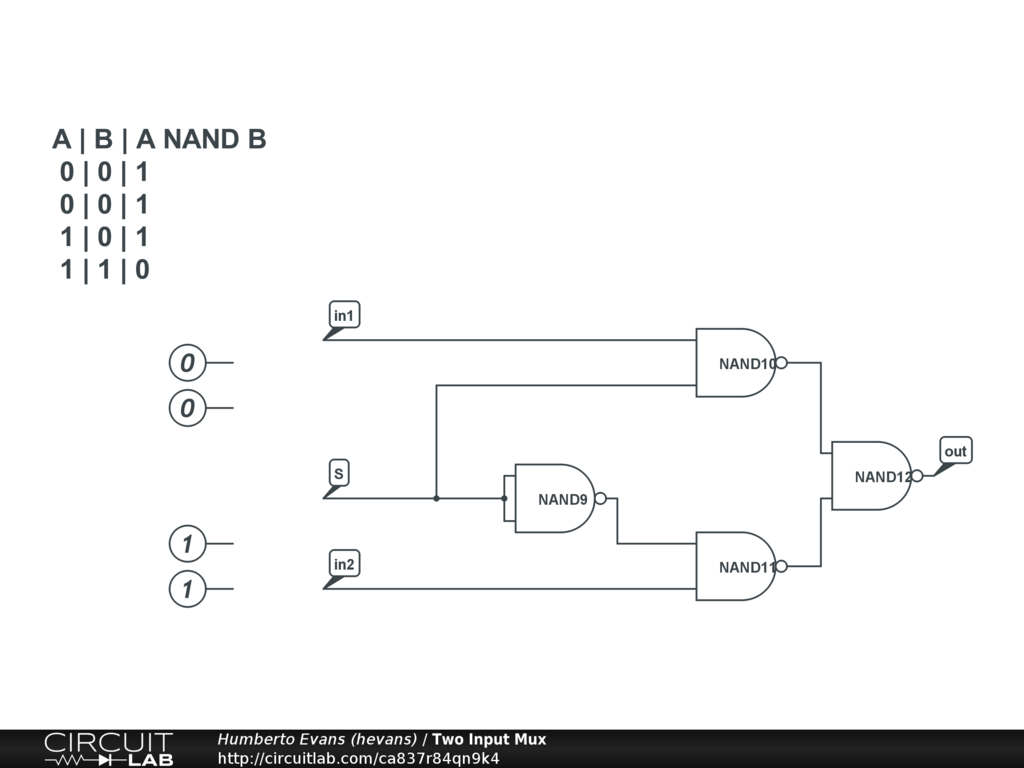## How to build and simulate a 2x1 multiplexer (MUX) from NAND gates

#### Jun 18 2020, 12:00 PM PDT · 1 comment »

In this video tutorial we build and simulate a two input 2x1 digital mux using only NAND gates.

## Circuit## Transcript

In this video we're going to build a two input multiplexer or two input digital mux made entirely out of NAND gates. So first what is a digital mux. A digital mux is a two input digital component that lets you select one of the two inputs based on the state of a third digital input.

CircuitLab has a digital mux component you can use, but that's not the point here, instead I'm going to be building my own entirely out of NAND gates.

So lets explore the NAND gate a little bit. Whenever I see a new component I like to simulate it just to see how it behaves. And the best way to do that is to just simulate it.

[Set up simulation with single NAND gate]

When the two inputs are digital low, the NAND gate makes the input high

Let's see what happens when I make one of these inputs a digital one. My V(out) is still high.

What happens when I make both inputs 1, now my V(out) is low. What I just went through is the truth table of a NAND gate.

[show truth table in CircuitLab]

That gives us a couple of very interesting properties. When one of the inputs is high, the output of a NAND gate is the opposite of the other input. I'm going to use that property later. Remember it.

The other property is that it's very easy to build an inverter using a NAND gate.

[set up NOT gate configuration]

So bear with me while I drop a whole bunch of gates into my circuit here.

[wire up the two input mux]

I'm going to name some nodes just to make it easier. This is my select node, and these two are going to be my input nodes. So what is going to happen in this configuration? What happens when I put a digital low at the select input. This puts a digital low at this NAND gate which means the output of this NAND gate is always high. Going back to the truth table, if any of the inputs are low the output is always high. So this in1 doesn't matter. The other thing this does, is because the output is always high then this nand gate is in that passthrough inverting configuration.

So lets go back to over here and see this NAND gate is a straight inverter here, which means this other NAND gate is also in this inverting pass through configuration. So in2 gets inverted twice, and ends up at the output. And we can see if I switch this select node to be a 1, then the opposite happens: in2 doesn't matter and in1 gets inverted twice at the output! We made a digital mux!